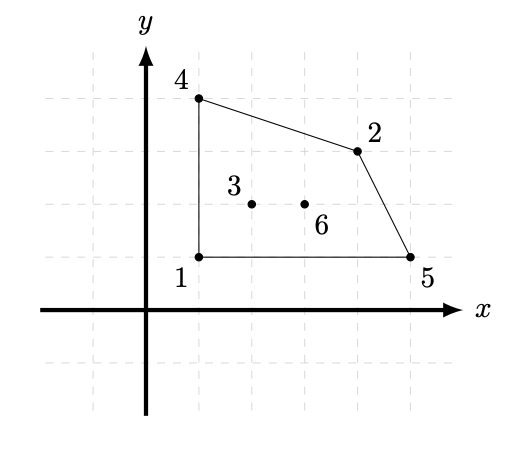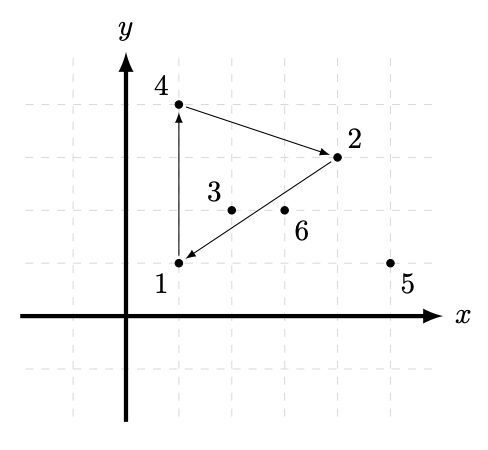시간 제한 메모리 제한 제출 정답 맞은 사람 정답 비율
2 초 512 MB 2 1 1 50.000%

## 문제

Byteland is a nice country with n (n ≥ 3) cities, represented as n distinct points on a 2D plane. The cities are numbered from 1 to n. As a tourist, you do not know the exact locations of the cities in Byteland. From a tourist magazine you learnt that no three cities are colinear.

The convex hull of a set of n points is a convex polygon with the smallest possible area such that all the n points are inside or on the border of this polygon. A convex polygon has all angles less than 180 degrees and cannot have self-intersections.

Your task is to find the number of vertices on the border of the convex hull of the set of Byteland cities. You may only ask questions for triples of different numbers of cities i, j, k (1 ≤ i, j, k ≤ n). Such a question concerns a triangle with vertices in cities i, j, k. The answer to the question indicates if traversing the vertices of the triangle in the order i, j, k is clockwise or counterclockwise.

## Communication

The library (trilib.h for C and C++) provides the following functions:

• int get_n();
Returns the number of cities.
• bool is_clockwise(int a, int b, int c);
Returns true if the vertices of the triangle a, b, c (1 ≤ a, b, c ≤ n, a ≠ b ≠ c ≠ a) are given in a clockwise order and false if they are given in the a counterclockwise order.
• void give_answer(int s);

For Java, the class trilib provides the following methods:

• static public int get_n();
• static public boolean is_clockwise(int a, int b, int c);
• static public void give_answer(int s);

After your program calls give_answer, it is not allowed to ask more questions. It should call give_answer exactly once.

In this problem, you are not allowed to read from the standard input or to write to the standard output.

After calling give_answer, your program should terminate immediately.

You may assume that the locations of points are established in advance and are not going to change during the execution of the program (that is, the library behaves in a completely deterministic way). For example, in the example test case (see below) calling give_answer(4) and immediately exiting would pass the test. Your program is allowed to try to guess the answer without being sure.

## 제한

• 3 ≤ n ≤ 40 000.
• You may call is_clockwise at most 1 000 000 times.

## 예제

Consider n = 6 cities located at (1, 1), (4, 3), (2, 2), (1, 4), (5, 1), (3, 2) as shown in the picture below. The convex hull is marked with lines. It contains four vertices on its border, so the result is 4.The following table shows an example interaction with a library that corresponds to this example.

Call Returned value
get_n() 6
is_clockwise(1, 4, 2)  true
is_clockwise(4, 2, 1) true
is_clockwise(1, 2, 4) false
is_clockwise(3, 6, 5) true
give_answer(4)

The picture below shows the triangle from the first query. The cities 1, 4, 2 are in a clockwise order, so the returned value is true.## 제출할 수 있는 언어

C++17, C++14, C++20, C++14 (Clang), C++17 (Clang), C++20 (Clang)

## 채점 및 기타 정보

• 예제는 채점하지 않는다.